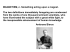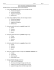# ELECTRICAL CIRCUITS

## Transcription

ELECTRICAL CIRCUITS
```5/5/2015
ELECTRICAL CIRCUITS
The CELL
The cell stores chemical energy and transfers it to
electrical energy when a circuit is connected.
When two or more cells are
connected together we call this a
Battery.
The cells chemical energy is used
up pushing a current round a
circuit.
S.MORRIS 2006
More free powerpoints at www.worldofteaching.com
What is an electric current?
simple circuits
An electric current is a flow of microscopic particles
Here is a simple electric circuit. It has a cell, a lamp
called electrons flowing through wires and
and a switch.
components.
+
cell
wires
switch
lamp
-
In which direction does the current flow?
from the Negative terminal to the Positive terminal of a cell.
To make the circuit, these components are connected
together with metal connecting wires.
simple circuits
When the switch is closed, the lamp lights up. This is
because there is a continuous path of metal for the
electric current to flow around.
If there were any breaks in the circuit, the current
could not flow.
circuit diagram
Scientists usually draw electric circuits using symbols;
cell
lamp
switch
wires
1
5/5/2015
Circuit Symbols:
Battery
Resistor
Light-bulb
Three general types of circuits:
Closed Circuit - There is a
complete loop with wires
going from one side of the
battery through a resistor(s)
to the other side of the
battery.
Switch
Open Circuit - There is not a
complete loop.
Wire
Short Circuit - There is a
complete loop, but it does
not contain any resistors.
Chapter 22: Current and Resistance
Only Working
Circuit
CURRENT
•Most modern day appliances require electricity to operate.
•The electricity generates a current which runs through the
appliance.
•The current is made out of charged particles
•Current is defined as the rate at which charge flows through a
surface area perpendicular to the direction of flow.
•For example: if the charges are flowing through a wire, the
area is the cross-sectional area of the wire
I
Q
t
Where: I = current (amps, A)
Q = change in charge or how much charge
flows (Coulombs, C)
t = time (seconds)
Example: The amount of charge that passes through the
filament of a certain lightbulb in 2.00 sec is 1.67 C. Find (a)
the current in the bulb and (b) the number of electrons that
pass through the filament in 5.00 sec.
I
Q 1.67C

 0.835A
t 2.00s
(b) First find the total charge in 5.00 sec. Then find how many
electrons that is (remember, 1 electron = 1.6x10-19C)
Q
5.00s
1electron
Q  4.175C 
 2.611019 electrons
1.6 10 19 C
0.835A 
Electric Current Flow
In real circuits it is electrons (-charges) that are
flowing. We call this kind of current “electron current”.
However, for simplicity we assume that all charge
carriers are positive. We draw current arrows in the
direction that such charges would move….that is, positive to
negative.
2
5/5/2015
Historically this is because Ben Franklin
guessed wrong about the charge
carriers in a metal being positive.
For an electric current to flow between two
points, two conditions must be met.
1. There must be a conducting path between the
points along which the charges can move.
WHY? The conducting material allows the
charges to move.
2. There must be a difference of electric potential
(volts) between the two points.
WHY? The potential difference makes the charges
want to move.
What does potential have to do with it?
But tip the pipe, increase the
potential of one end so there is a
difference in potential across the
ends of the pipe, and water will
flow.
And so it is with
electric current flow.
Water doesn’t flow in the pipe when both ends
are at the same height. That is to say, water will not
flow when both ends of the pipe are at the same
potential (height).
You get current to flow through a conductor by applying
a voltage across it. This voltage creates an electric field within
the conductor. This electric field in turn creates a force on all
the charges within the conductor. If charges are free to move,
as in a conductor, they do. In solids these charges are always
electrons.
An ampere is a fairly large amount of current:
0.1 A flowing between your hands across your
heart will kill you. (Fortunately, your body has
fairly high resistance so it takes a substantial
voltage to drive that much current.)
Current flow is the measure of electrons moving within a
conductor.
Amperes, or amps, measures the amount of current that flows.
Amps is named after a 19th-century French physicist, AndreMarie Ampere, and abbreviated A.
-
+
3
```

### Current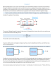### www.chlorofil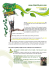### Electricity Study Guide - Key What are series circuits?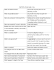### File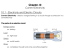### Assignment 1- Unit 35 Activity 1 P1 P2 P4 M1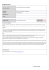### From the Desk of Dean G. De Vries Bock - ELCSA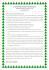### Vincent Camus